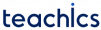# Capacitive Transducers: Construction, Working principle and Advantages

A capacitive transducer is a passive transducer that works on the principle of variable capacitances. It is used to measure physical quantities such as displacement, pressure, etc.

## Construction of capacitive transducer

A capacitive transducer contains two conducting parallel metal plates separated by a dielectric medium.

## Working Principle of capacitive transducer

The capacitance between these two plates can be expressed asWhere ϵ is the permittivity of the medium, A is the area of the plates and d is the distance between two plates.

The capacitance of the transducer is measured using the bridge circuit. The output impedance of the transducer is given byWhere C is the capacitance and f is the frequency of excitation. So a capacitive transducer can be used to measure the mechanical vibrations.

The capacitance between two plates can be varied by any of the following methods.

• By changing the distance between two plates (d)
• By changing the permittivity of the dielectric medium (ϵ)
• By changing the area of overlapping of plates (A)

### By changing the distance between two plates

The capacitance can be varied by changing the distance between two plates. From the equation for C, we can observe that C and d are inversely proportional to each other. That is, the capacitance value will decrease with increasing distance and vice-versa. This principle can be used in a transducer by making the left plate fixed and the right plate movable by the displacement that is to be measured as shown in the figure.

The change in distance between two plates will vary the capacitance of the transducer. Change in capacitance can be calibrated in terms of the measurand. These types of transducers are used to measure extremely small displacements.

The distance capacitance curve is shown in the figure.

### By changing the permittivity of the dielectric medium

Another method to change the capacitance value is by changing the permittivity of the dielectric material (ϵ). The permittivity and capacitance value are directly proportional to each other.

In this arrangement, a dielectric material is filled into the space between the two fixed plates. It can be moved using the arm. This causes a variation in dielectric constant in the region. The change in dielectric constant will vary the capacitance of the transducer.

### By changing the area of overlapping of plates

The capacitance can also be changed by varying the area of overlapping of plates.

As shown in the figure, one plate is kept fixed and the other movable. When the plate is moved, the area of overlapping of plates changes, and the capacitance also changes. The capacitance value and area are directly proportional to each other. These types of transducers are used to measure relatively large displacements.

The distance-capacitance curve is shown in the figure.

• Sensitivity is high.
• Requires small power to operate.